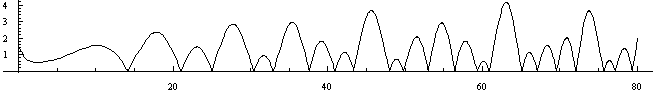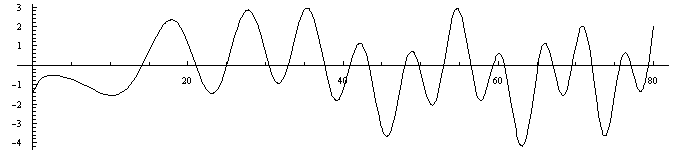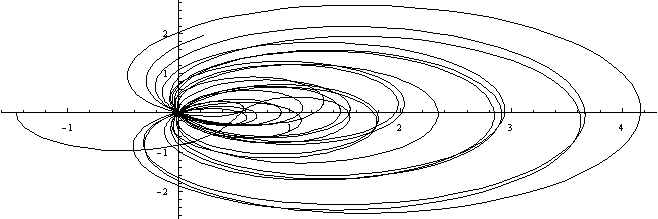## Ten Trillion Zeta Zeros

Ed Pegg Jr., October 18, 2004

I've been watching the progress of Zetagrid.net, waiting for the trillionth nontrivial zero of the Zeta function to be calculated. As I write, they have calculated "935.7 billion nontrivial zeros of the Riemann zeta function in 1146 days." I thought I'd have a nice little story next month once they went over a trillion. Instead, I got a major surprise from an unexpected direction.

What is the Zeta function, ζ(s)? Very simply, ζ(s) = Σ (1/n)s = (1/1)s + (1/2)s + (1/3)s + (1/4)s + (1/5)s + (1/6)s + (1/7)s + ... an infinite sum of fractions. If you look at ζ(1), and start adding fractions, you might suspect that it eventually hits some upper limit. The first 10 sums are {1, 3/2, 11/6, 25/12, 137/60, 49/20, 363/140, 761/280, 7129/2520, 7381/2520}or {1., 1.5, 1.83333, 2.08333, 2.28333, 2.45, 2.59286, 2.71786, 2.82897, 2.92897}. The fractions up to a googol (10100) sum to about 230.835. There is no upper limit to this sum. Euler showed that the first n terms equals approximately Log[n] + γ, where γ is Euler's constant, about 0.57721566490153286061. Lorenzo Mascheroni found the first 19 digits of this constant.

What is (1/1)2 + (1/2)2 + (1/3)2 + (1/4)2 + (1/5)2 + ... ? For decades, ζ(2) = Σ (1/n)2 was known as the Basel problem, and stumped the world's mathematicians. At the age of 28, in 1735, Euler proved that ζ(2) = π2/6, a result which brought him instant fame. In addition, he proved ζ(4) = π4/90, ζ(6) = π6/945, ζ(8) = π8/9450, ζ(10) = π10/93555, ζ(12) = 691π12/638512875, all the way up to ζ(26). For even n, it turns out that 2 ζ(n) = |Bn|(2π)n/n!, where Bn is the nth Bernoulli number. For odd n, little is known, beyond a proof by Apery that ζ(3) is irrational.

Euler further showed ζ(s) = Σ (1/n)s = Π(1 - p-s)-1, or (1/(1-(1/2)s) × (1/(1-(1/3)s) × (1/(1-(1/5)s) × (1/(1-(1/7)s) × (1/(1-(1/11)s) × ..., which relates the Zeta function to the primes.

In 1859, Bernhard Riemann took a look at ζ(s) = Σ (1/n)s = Π(1 - p-s)-1 when s was a complex number. He used the Zeta function to describe the distribution of primes. In addition, he noted his suspicion that all of the nontrivial solutions for ζ(s)=0 seemed to be of the form ½ + βi. This became known as the Riemann Hypothesis.

We can look at |ζ(½ + βi)| for β from 0 to 80, as a test of the hypothesis. It seems Riemann was correct, starting out.Figure 1: Plot[Abs[Zeta[1/2 + I t]], {t, 0, 80}]

Here is a similar function, RiemannSiegelZ[t], for t from 0 to 80. Note how it's the save as the above, with alternate humps flopped over. After 10, if it ever failed to hit the zero line as it rose and fell, the Riemann Hypothesis would be false.Figure 2: Plot[RiemannSiegelZ[t], {t, 0, 80}]

We can also direction plot the complex numbers resulting from ζ(½ + βi) for β from 0 to 80. The path returns continuously to {0,0}. After 10, if the curve ever missed the origin, the Riemann Hypothesis would be false.Figure 3: zeta[s_] := {Re[temp = Zeta[N[s]]], Im[temp]}
ParametricPlot[zeta[1/2 + I t], {t, 0, 80}]

As results extended farther and farther, ζ(½ + βi) continued to be the source of all nontrivial zeroes, without exception. No-one has managed to prove to the satisfaction of the mathematical community that ζ(α + βi)=0 implies α=½ or β=0. The Clay Institute has offered a million dollar prize to the first proof that passes all scrutiny.

Zetagrid.net offers \$1000 for the first counterexample. As I mentioned, they calculated the first 935.7 billion nontrivial zeros.

Xavier Gourdon used the algorithm of Odlyzko and Schönhage to calculate the first ten trillion (10,000,000,000,000) nontrivial zeros. He started work on the problem back in 1998, when he wrote a non-optimized program to check the results known for the first 1.5 billion zeros. Later, he realized he could improve his program, and did so. He has offered his code to the Zetagrid project, created a site detailing the results, and written a paper. Below is his table detailing the history of the problem in terms of known Zeta zeros.

 Year n Author 1903 15 J. P. Gram  1914 79 R. J. Backlund  1925 138 J. I. Hutchinson  1935 1,041 E. C. Titchmarsh  1953 1,104 A. M. Turing  1956 15,000 D. H. Lehmer  1956 25,000 D. H. Lehmer  1958 35,337 N. A. Meller  1966 250,000 R. S. Lehman  1968 3,500,000 J. B. Rosser, J. M. Yohe, L. Schoenfeld  1977 40,000,000 R. P. Brent  1979 81,000,001 R. P. Brent  1982 200,000,001 R. P. Brent, J. van de Lune, H. J. J. te Riele, D. T. Winter  1983 300,000,001 J. van de Lune, H. J. J. te Riele  1986 1,500,000,001 J. van de Lune, H. J. J. te Riele, D. T. Winter  2001 10,000,000,000 J. van de Lune (unpublished) 2004 900,000,000,000 S. Wedeniwski  2004 10,000,000,000,000 X. Gourdon and Patrick Demichel 
Figure 4. History of numerical verification of the RH (by Xavier Gourdon)

So far, no counterexamples. Whew! A counterexample would have shattered the current knowledge on the distribution of primes. For an excellent demonstration on the relationship between prime numbers and the Zeta zeros, see Prime Number Races by Andrew Granville and Greg Martin.

In 1973, Hugh Montgomery and Daman Chowla were having tea. Freeman Dyson was across the room having a snack of his own. Against protests, Chowla dragged his colleague over to meet Dyson, who greeted them cordially. When asked what he was studying, Montgomery answered that the distribution of Zeta zeros seemed to be about 1 - [sin(πu)/(πu)]2, that's what he was currently looking at. Dyson responded, "Well, that's the density of the pair correlation of eigenvalues of random matrices in the Gaussian Unitary Ensemble." During a short tea break, Dyson had connected quantum physics with the Riemann hypothesis. This connection is now called the GUE Hypothesis. Xavier's results adds strength to that hypothesis.

If you'd like to experiment with the Riemann hypothesis yourself, The Mathematical Explorer has a wonderful introduction written by Stan Wagon. You can also get a nice Zeta function poster.

Gourdon has many more results from his goldmine of data, it's all in his paper. Once Zetagrid gets ramped up to the new methods, it might become possible to use massive databases of Zeta zeros to pinpoint new large primes.

References:

Xavier Gourdon, "Computation of zeros of the Zeta function", http://numbers.computation.free.fr/Constants/Miscellaneous/zetazeroscompute.html.

Xavier Gourdon, "The 1013 first zeros of the Riemann Zeta function, and zeros computation at very large height", 2004. http://numbers.computation.free.fr/Constants/Miscellaneous/zetazeros1e13-1e24.pdf.

Andrew Granville and Greg Martin, "Prime Number Races," 2004. http://www.arxiv.org/PS_cache/math/pdf/0408/0408319.pdf.

MacTutor History of Mathematics, Leonhard Euler. http://www-history.mcs.st-andrews.ac.uk/Mathematicians/Euler.html.

MacTutor History of Mathematics, Lorenzo Mascheroni. http://www-history.mcs.st-andrews.ac.uk/Mathematicians/Mascheroni.html.

MacTutor History of Mathematics, Bernhard Riemann. http://www-history.mcs.st-andrews.ac.uk/Mathematicians/Riemann.html.

Andrew Odlyzko, "On the Distribution of Spacings Between Zeros of the Zeta Function," Math. Comp., 48 (1987), pp. 273-308. http://www.dtc.umn.edu/~odlyzko/doc/zeta.html.

Ed Pegg Jr, "The Möbius Function", 2003. http://www.maa.org/editorial/mathgames/mathgames_11_03_03.html.

Matthew Watson, "Dyson and Montgomery's fortuitous meeting over tea", http://www.maths.ex.ac.uk/~mwatkins/zeta/dyson.htm.

Eric W. Weisstein. "Bernoulli Number, Riemann Hypothesis," From MathWorld--A Wolfram Web Resource. http://mathworld.wolfram.com/.